Next: Multi-Time Step Nodal Subcycling Up: 1-D ANALYSIS AND RESULTS Previous: 1-D ANALYSIS AND RESULTS   Contents

Problem Description

Typical 1-D problems involve bars or beams under axial loading. In order to test our various algorithms we define a reference problem composed of a beam fixed at one end and free and the other with an applied axial load at the free end, as seen in Figure 3.1. We use this general problem for all of our analyses in the upcoming sections.This beam, of length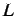, is fixed at the right end and has a compressive axial force applied on the left, corresponding to an axial stress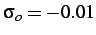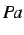. The beam is homogeneous and has a cross-sectional area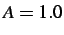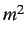, density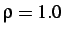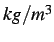and Young's modulus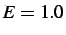. The beam wave speed given by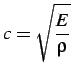(3.1)

is thus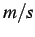.

In 1-D the analytical solution to a beam problem can be obtained by solving the wave equation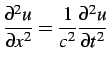(3.2)

with initial conditions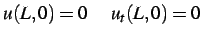(3.3)

and boundary conditions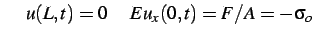(3.4)

where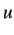is the displacement and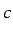is the wave speed.

The solution to this problem is represented by the x-t (displacement-time) diagram in Figure 3.2. The displacement, velocity and stress profiles, for a sample point at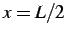, are shown in Figure 3.3.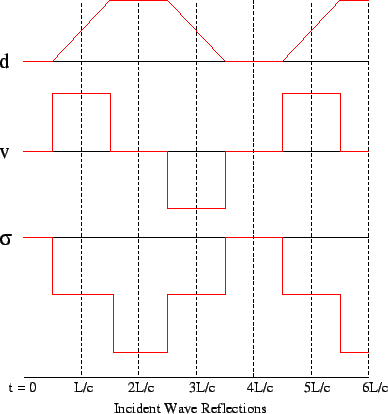Next: Multi-Time Step Nodal Subcycling Up: 1-D ANALYSIS AND RESULTS Previous: 1-D ANALYSIS AND RESULTS   Contents
Mariusz Zaczek 2002-10-13